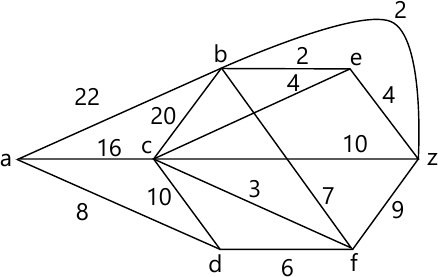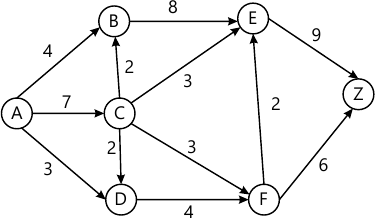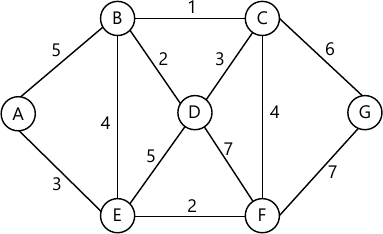MORE IN Discrete Structures
SPPU Information Technology (Semester 3)
Discrete Structures
December 2015
Total marks: --
Total time: --
INSTRUCTIONS
(1) Assume appropriate data and state your reasons
(2) Marks are given to the right of every question
(3) Draw neat diagrams wherever necessary

Solve any one question from Q1 and Q2
1 (a) (i) Show that (A-B)-C=A-(BUC) using Venn diagram.
3 M
1 (a) (ii) Obtain CNF for the following ¬(P ∨ q) ↔ (p ∧ q).
3 M
1 (b) Draw the Hasse diagram of relation R on A. Let A = {1, 2, 3, 4, 5} and R = {(1, 1), (2, 1), (2, 2), (3, 1), (3, 2), (3, 3), (4, 1), (4, 2), (4, 3), (4, 4), (5, 1), (5, 2), (5, 3), (5, 4), (5, 5)}
6 M

2 (a) Suppose that 100 out of 120 mathematics students at a college take at least one of the languages French, German and Russian. Also suppose
65 study French
45 study German
42 study Russian
20 study French and German
25 study French and Russian
15 study German and Russian
i) Find the number of students who study all the three languages.
ii) Find incorrect of students in each region of Venn diagram.
iii) Determine the number K of students who study:
a) Exactly one language
b) Exactly two language.
6 M
2 (b) Let A = {a, b, c, d}, Let R be a relation on set A whose relation is R={ (b, a), (c, b), (a, d), (b, d), (c, d)}. Find transitive closure using Warahall's method.
6 M

Solve any one question from Q3 and Q4
3 (a) G={0, 1, 2, 3, 4, 5, 6, 7} and operation is '+S' addition modulo8, then (G, +8) is an abelian group.
6 M
3 (b) Find the shortest path from a to z using Dijkstra's Algorithm.6 M

4 (a) Define the following terms with suitable example:
i) Factor of Graph
ii) Weighted Graph
iii) Graph Coloring
iv) Bipartite Graph
6 M
4 (b) Find the minimum distance of an encoding function e: B2→B5 given as: e(2, 5)
e(0, 0) = 0 0 0 0 0
e(0, 1) = 1 0 0 1 1
e(1, 0) = 0 1 1 1 0
e(1, 1) = 1 1 1 1 1
6 M

Solve any one question from Q5 and Q6
5 (a) Construct a binary tree from given inorder and preorder traversals:
 Inorder    : A E B D C F G K I H J L Preorder  : F E A D B C G H I K J L
6 M
5 (b) Find the maximum flow in the following transport network:7 M

6 (a) Determine the minimum spanning tree using Prims algorithm for the following graph:6 M
6 (b) For the following set of weight, construct the optimal binary prefix tree. For each of the weight in the set, give the corresponding prefix code:
1, 2, 4, 5, 6, 9, 10, 12, 15
7 M

Solve any one question from Q7 and Q8
7 (a) If 2 cards are drawn from a usual deck of well suffled pack of 52 cards, what is the probability that 2 aces are drawn?
3 M
7 (b) Two fair dice are rolled, what is the probability that:
i) Sum of the faces is a perfect square.
ii) Sum of the faces is neither 5, 6 or 7.
4 M
7 (c) In how many ways can 10 boys and 5 girls stand in a line so that no two girls are next to each other? (All boys and girls are distinct).
ii) In how many ways can 10 boys and 5 girls stand around a circle so that no two girls are next to each other? (All boys and girls are distinct).
6 M

8 (a) A bag contains 5 red, 4 white and 8 blue balls. 4 balls are drawn at random. What is the probability that there is at least one ball of each colour?
7 M
8 (b) A student must answer 7 out 10 questions in an examination.
i) How many choices does the student have?
ii) How many choices does she have if she must answer the first three questions?
iii) How many choices does she have if she must answer at least three of the first five questions?
6 M

More question papers from Discrete Structures